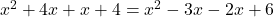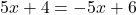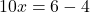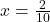Amin is attempting to solve the following equation. (x+1)(x+4)=(x-2)(x-3) solve the equation

Question

Amin is attempting to solve the following equation. (x+1)(x+4)=(x-2)(x-3) solve the equation

in progress 0
6 months 2021-08-04T12:14:09+00:00 2 Answers 20 views 0

1. Step-by-step explanation:

Hey there!

Given;

(X+1) (X+4) = (x-2) (x-3)

Multiply (X+1) and (X+4) on left side and (x-2) and (x-3) on right side.Simplify it.Therefore, x= 1/5.

Hope it helps....